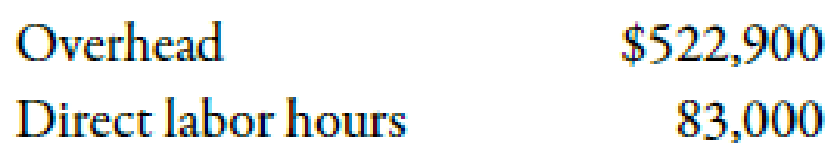Chapter 4, Problem 38E### Managerial Accounting: The Corners...

7th Edition
Maryanne M. Mowen + 2 others
ISBN: 9781337115773

#### Solutions

Chapter
Section### Managerial Accounting: The Corners...

7th Edition
Maryanne M. Mowen + 2 others
ISBN: 9781337115773
Textbook Problem
21 views

# Calculating the Predetermined Overhead Rate, Applying Overhead to ProductionAt the beginning of the year, Debion Company estimated the following:Debion uses normal costing and applies overhead on the basis of direct labor hours. For the month of March, direct labor hours were 7,600.Required: 1. Calculate the predetermined overhead rate for Debion. 2. Calculate the overhead applied to production in March.

1.

To determine

Calculate the predetermined overhead rate for Company D.

Explanation

The amount which is calculated at the beginning of the accounting year for a related activity by dividing the total estimated annual overhead by estimated annual activity level is known as the predetermined overhead rate.

Use the following formula to compute the predetermined overhead rate for Company D:

2.

To determine

Calculate the overhead applied to production in March.

### Still sussing out bartleby?

Check out a sample textbook solution.

See a sample solution

#### The Solution to Your Study Problems

Bartleby provides explanations to thousands of textbook problems written by our experts, many with advanced degrees!

Get Started